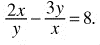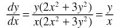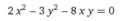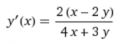# Implicit differentiation.

#### hsazerty2

Joined Sep 25, 2015
25
Hello,
we have the following equation (pair of intersecting lines without the origin) :using implicit differentiation, we find :Now, if we reorganize the terms of the equation, we get:using implicit differentiation, we find :Why is the result different from the first one ?
If we only reorganize the terms, the plot of the equations should be the same (except at the origin), so the derivative at any point (slope of tangent) shoud be the same, no ?

#### Papabravo

Joined Feb 24, 2006
12,405
I'm going to take a wild guess and say that you've made a mistake somewhere. Not only can the lines not include the origin, the can't include either axis as well, at least in the first formulation since we get something very large being equal to 8. I get the same answer you did for the second method.

Last edited:

#### minutepapillon

Joined Jun 2, 2017
8
Hi,
I make mistakes so don't 100% trust what i say ... but In your first calculation in the numerator, I get 3y and not 3y² and this kills your simplification.

Sorry if I am wrong ...
Hope this helps.

#### Tesla23

Joined May 10, 2009
374
Check when these two expressions are equal:

$$\frac{y}{x} = \frac{2(x-2y)}{4x+3y}$$

and simplify:

$$2x^2 - 3y^2 - 8xy = 0$$

i.e. these two expressions are equal on your curve. What's the problem?

#### minutepapillon

Joined Jun 2, 2017
8
Sorry, I made a mistake ! (shame on me ...)

#### MrAl

Joined Jun 17, 2014
6,500
Hello,
we have the following equation (pair of intersecting lines without the origin) :
View attachment 127902
using implicit differentiation, we find :
View attachment 127903

Now, if we reorganize the terms of the equation, we get:
View attachment 127904
using implicit differentiation, we find :
View attachment 127905
Why is the result different from the first one ?
If we only reorganize the terms, the plot of the equations should be the same (except at the origin), so the derivative at any point (slope of tangent) shoud be the same, no ?
Hi,

I think you may have made a mistake, but it would help if you did three things:
UPDATE: You just left out the last step.
(see far below for corrections)

1. Show the equation for the two lines that you say are intersecting and graph them if possible (the two equations would be good alone too though).
2. Show exactly the steps you took to perform the first implicit differentiation.
3. Tell us what the end application is. Knowing what this implicit differentiation is going to be used for may tell us which solution we should use.

If you start from two lines and then try to find the intersection of those two lines, then what is the purpose of finding the derivative?

Also, when you multiply both sides of an equation by a variable, you may introduce new solutions.
For example, the equation:
2*x^2+x=0

has two solutions:
x=-1/2, x=0

but divide both sides by x and we get:
2*x+1=0

which has only one solution:
x=-1/2

Corrections:
The first time i did this i did it the wrong way too. The key point i think is that when we take derivatives of functions we have to follow more complicated rules than when we do just single variable terms. For example, the derivative of:
y/x

is not y'/x', it is:
(x*y'-y*x')/x^2

and this goes even when we do implicit differentiation as would the following:
d/dx (x*y) is not equal to x'*y' it is equal to x*y'+y*x'.

Try following those rules and see what you get.

As the last step, you have to solve for y in the original solution and then substitute that into the derivative. That solution should match the derivative found after solving for y and then taking the derivative of y with respect to x.

Try doing a simpler one first such as:
x^2+y^2=25

and see what you get for dy/dx. Keep in mind that there may be more than one solution too.

Last edited: